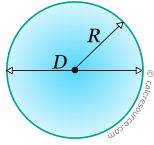## Properties of a Circular Area

This tool calculates the basic geometric properties of a circular area. Enter the shape dimensions R or D below. The calculated results will have the same units as your input. Please use consistent units for any input.

 R = ...or D = Geometric properties: Radius = Diameter = Area = Perimeter =## Definitions

### Geometry

The circle is a curved, closed, planar shape, every point of which has equal distance from a constant point, called centre. The points (x,y) of the circumference, satisfy the equation:

where x0, y0the coordinates of the centre of the circle and R its radius.